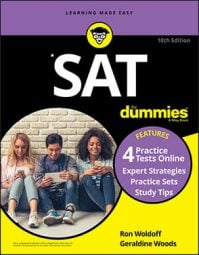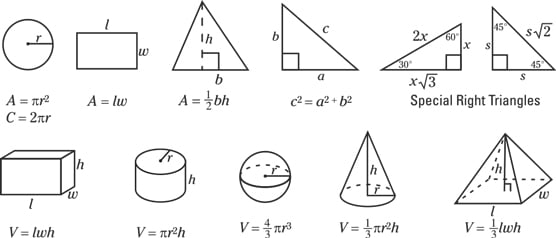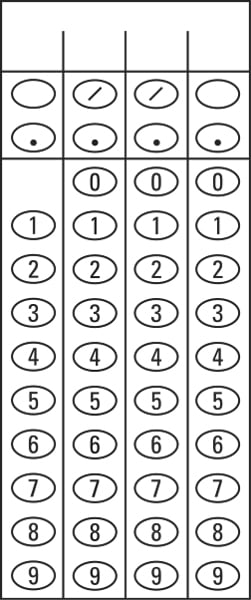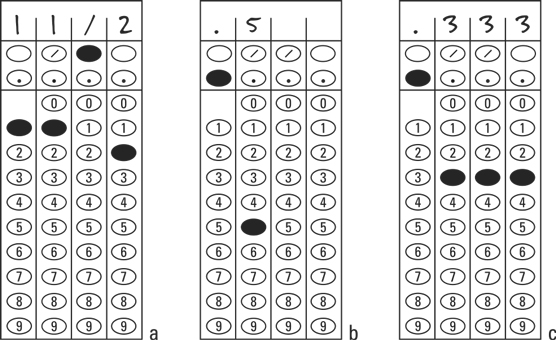##### SAT For DummiesThere are two math tests on the SAT, back to back. The first math test features 20 questions for 25 minutes, with no calculator allowed. The second math test has 38 questions for 55 minutes, and you are allowed to use a calculator. Other than that, the two tests are basically the same. One thing to note is that the questions tend to start out at a low level of difficulty, which increases as you progress through the test.

Difficulty is relative. A question that’s easy for you may be challenging for your friend, and vice versa. It doesn’t matter anyway, because if you know how to approach the question, it’s easy no matter what. The SAT determines difficulty by the number of students who missed the question during a trial, not by the question itself. So, a “difficult” question to the SAT is simply one where more students didn’t know how to approach it. If you know how to approach the question, the question is easy.

Furthermore, if you know how to approach an SAT math question, then it takes less than a minute to answer. This means that if you know what to do, you can answer all of the questions in the SAT math tests easily and without rushing. And this leads to the first two bits of wisdom:

• Make each question easy by knowing what you’re doing. There aren’t that many topics on SAT Math. There are a few, and they’re all topics that most students see in high school. There are plenty of math topics that you don’t see, such as matrices, which only appear on the ACT.
• Don’t rush, because you’ll make all kinds of mistakes. Instead, to speed up your progress through the test, make sure you don’t get stuck. The way you don’t get stuck is by knowing what you’re doing. Then you’ll answer all the questions easily with time to spare.
Here are more bits of wisdom (also known as Math Test Strategies) along those same lines:
• Don’t take more than a minute on any one question. If you don’t know what to do and you get stuck, that’s okay. Take a guess and move on:
• Circle the question in your booklet.
• Fold the corner of the page in your booklet.
• Move on to the next question.
• Come back to this question at the end of the section.
• If you find yourself working a lot of math, you missed what the question is asking. An SAT Math question is more like a puzzle than a math problem. It never takes a lot of math, but it may take a strategic approach. If you understand the question and set it up correctly, all the fractions cancel and everything lands nicely and neatly. If you don’t understand what it’s asking, you start working a lot of math, so step back and follow the preceding strategy: Take a guess, move on, and come back to this question at the end of the section. You’ll probably spot how to work the question on this second look.
• Treat each question like it’s worth the same number of points. A question that goes fast is worth the same points as one that takes forever. So, work the fast questions first! Then go back to the time-consumers.
• Use the test booklet as your scratch paper. Write your calculations in the extra blank space, but take time to bubble in your answers. Even though the proctor collects the test booklet, your notes and figuring don’t affect your score.
• If you’re almost out of time, bubble 'til the end. Really, this shouldn’t happen. Worst case, take the plunge and guess through the end of the test. A wrong answer is no worse than an unanswered one, so you may as well take a shot at getting it right.
• Bubble your answer after each question. Don’t wait until the end and then go back and bubble them all. What could possibly go wrong?

## Formulas on the SAT math tests

The first page of each math test has a set of formulas to help you solve the problems. By the time you’re on the second page of the test, you’ll probably forget it’s there — almost everyone does — but these formulas, shown, are still good to know.The SAT gives you these formulas.

## Just gridding

Of the 58 math test questions, most are good ol' multiple choice, but 13 (five in the first math test and eight in the second) ask you to provide your own answers and bubble them into a grid. These questions are known as grid-ins. This figure shows a sample blank grid-in.A blank grid-in

The grid-in problems are normal math questions but with certain rules:

• You can’t grid in negative numbers. No answer is negative, so each answer must be positive or zero.
• Don’t grid in a mixed number. If you come up with 5-1/2 as your answer, don’t grid in 51/2, because the scanner will read “51 over 2,” not “five and one-half.” Instead, convert your answer to an improper fraction. Grid in 11/2 (11 over 2), as shown, or grid in a decimal: 5.5.
• You can start from the left, right, or middle. Just be sure that you have enough boxes for the whole answer.
• If there is more than one correct answer, pick one and go with it. If it turns out that the answer can be either 4 or 5, just pick one and grid it in. Don’t worry about the other answer: The system will accept either one. That also goes for a range: If your answer is between 3 and 7, then any number between 3 and 7 is considered correct.
• Check whether the answer needs to be rounded. If you find that the answer is between 6 and 12, check and see whether the answer must be an integer. A lot of times when the answer is a decimal, the question specifies that it should be rounded to a certain number of decimal places.

For example, if you correctly compute an answer of 1.75, and the question specifies that the answer needs to be rounded to one decimal place, then a gridded answer of 1.75 is considered incorrect, while the rounded answer of 1.8 is considered correct.

• Don’t place zeroes before a decimal point. If your answer is .5, darken the oval for the decimal point and the five, as in .5, not 00.5.
• If your answer is a repeating decimal, fill in all the boxes, rounding off the last number only. In other words, darken the ovals for .333 or .667 ( 1/3 and 2/3 expressed as decimals), not .3 or .67 (See C in the figure). Note that you don’t have to round the last number: If the answer is 2/3, you can grid that, or .666 or .667: any of those three is correct. Probably just grid the fraction.Three grid-ins, properly filled in

Don’t worry if you get more than one possible answer. Some grid-in questions have several possible right answers. (Usually those problems read something like, “what is one possible value of . . .”) Just pick one answer and you’re set.

## Get familiar with the math tests

Worried? Don’t be. The most important thing to know — that phrase is used a lot, but it’s always true — is that certain math topics are on the SAT, and certain math topics aren’t. If you know what these topics are, and the way that the SAT asks about them, then each answer in the math tests is within your reach. These topics are listed here.

The SAT throws the occasional curveball question in its math tests. There may be some unusual topic just on the edge or outside the scope of SAT Math. These are few and far between, but if you encounter one, you know what to do: Circle it, take a guess, and come back to it later. If you know how to answer most of the math questions, you can answer them quickly and have time left over to focus on the harder questions. That means you.

• Numbers and operations: These are about a quarter of the math test questions. Basically, anything that doesn’t involve an x or a drawing falls into this category, which includes whole number operations, fractions, ratios, exponents, and radicals. Of these, ratios and exponents are typically the most commonly occurring.
• Algebra and functions: These are just under half of the math test questions and include anything with an x or any other letter that represents a number. The most commonly occurring algebra questions include linear equations, systems of equations (which are basically two linear equations), and parabolas.
• Geometry and trigonometry: These are about a quarter of the math test questions and include the typical circles and trapezoids along with 3-D shapes, such as cubes and cylinders. There’s some subtracting the areas or volumes of shapes, and just a little trig. The most common questions in this group involve triangles and parts of circles (such as half of a wheel) along with trig.
• Statistics and probability: These are just a few of the math test questions and involve averages and graphs, including scatterplots. The most common questions in this group are definitely the graphs.
So, there you are. You now know the secrets of the SAT math tests. Nice! Note that whether a group of questions is about a quarter or half of the Math Test questions, or whether certain topics are more common than others, varies from exam to exam. The preceding assessments, along with the estimated priority of each math topic, are based on the review of countless SATs with students. Each exam is different, and yours may not have a quarter of this question type or half of that one. But it’ll be close.

Also, unlike the Reading Test and Writing and Language Test, where you learn strategies to take on 11 questions at a time, the math test strategies focus on one question at a time.